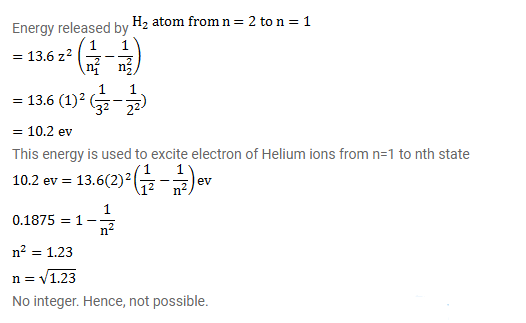# Radiation coming from transition n=2 to n=1

Question:

Radiation coming from transition $n=2$ to $n=1$ of hydrogen atoms falls on helium ions in $n=1$ and $n=2$ states. What are the possible transitions of helium, ions as they absorb energy from the radiation?

Solution: